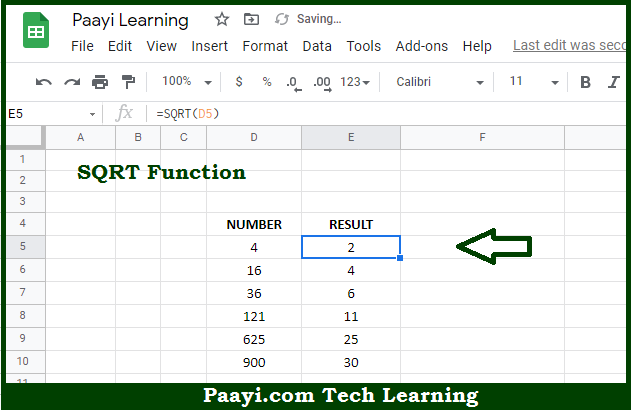# Learn How to Use Google Sheets SQRT Function

Written by | 0 Comments | 631 Views

In this article, you will learn how to use the Google sheets SQRT function and its prime function in Google sheets. You will also get to know the Google sheets SQRT function return value and syntax with the help of some examples.

The main function of the Google Sheets SQRT function is to get the positive square root of the given number. That implies, with the help of the SQRT function you can able to return the square root of the positive number. It should be noted that SQRT will return the #NUM error if the number is negative. If you want to get the square root of a negative number, you need to use the ABS function as = SQRT(ABS(A1)). So, with the help of the SQRT function, you can able to get the positive square root of the given number.

Return Value of SQRT Function

The return value will be the positive square root.

Syntax of SQRT Function

=SQRT(number)

Where the arguments:

• number: This is the number of which you want to get the square root.

## How to Use Google Sheets SQRT Function?So we know that Google Sheets SQRT function you can able to get the positive square root of the given number. That implies, with the help of the SQRT function you can able to return the square root of the positive number. It should be noted that SQRT will return the #NUM error if the number is negative. If you want to get the square root of a negative number, you need to use the ABS function as = SQRT(ABS(A1)). So, with the help of the SQRT function, you can able to get the positive square root of the given number.